0
577

Geometry is an important topic in the Quant section of the SNAP Exam. You can also download this Free Geometry Questions for SNAP PDF (with answers) by Cracku. These questions will help you to practice and solve the Geometry questions in the SNAP exam. Utilize this PDF practice set, which is one of the best sources for practising.

Question 1: If a 30 meter ladder is placed against a wall such that it just reaches the top of the wall, if the horizontal distance between the wall and the base of the ladder is 1/3rd of the length of ladder, then the height of wall is :

a) 25 meter

b) $20\sqrt{2}$ meter

c) $20\sqrt{3}$ meter

d) 20 meter

Solution: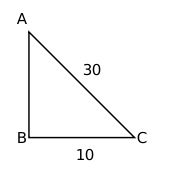Given AC = 30 m and BC = $\frac{1}{3}AC$ = $\frac{1}{3}\left(30\right)$ = 10 m

Applying pythagoras theorem,

$AB^2+\ BC^2=\ AC^2$

$AB^2$ = $30^2-10^2$ = 800

AB = $20\sqrt{\ 2}$

Question 2: A copper wire having length of 243m and diameter 4 mm was melted to form a sphere. Find the diameter of the sphere :

a) 17 m

b) 18 cm

c) 15 cm

d) 20 cm

Solution:

Volume of copper wire melted will be equal to volume of sphere.

Volume of copper wire = Area $\times\$ length = $\pi\ \left(\frac{2}{10}\right)^2\times\ 24300\ cm^3$

Let the radius of sphere be r

$\frac{4}{3}\pi\ r^{3\ \ }=\ \pi\ \left(\frac{2}{10}\right)^2\times\ 24300\ cm^3$

$r^3$ = 729

r = 9 cm

Diameter of sphere = 2(9) = 18 cm

Question 3: If a cuboidal box has height, length and width as 20 cm, 15 cm and 10 cm respectively. Then its total surface area is__________.

a) 1300$cm^{2}$

b) 1400$cm^{2}$

c) 1200$cm^{2}$

d) 1100$cm^{2}$

Solution:

Total surface area = 2(lb + bh + hl) = 2(150 + 200 + 300) = 1300 $cm^2$

Question 4: Angle bisectors of a parallelogram form a _____.

a) parallelogram

b) square

c) rhombus

d) rectangle

Solution: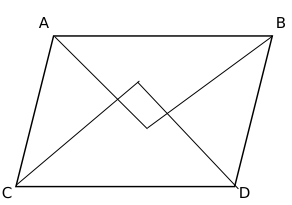Angular bisectors from A and B intersect at P, B and D intersect at Q, C and D intersect at R, and A and C intersect at S.
In a parallelogram ABCD, AC is parallel to BD.
$\angle\ CAB\ +\angle\ ACD\ =\ 180^{\circ\ }$
$\angle\ CAS\ +\angle\ ACS\ =\ 90^{\circ\ }$
In triangle CAS,
$\angle\ ASC\ =\ 180^{\circ\ }-90^{\circ\ }=90^{\circ\ }$
As all the angles of PQRS are $90^{\circ\ }$, PQRS is a rectangle.

Question 5: A tree bends due to heavy storm and now its peak touches the ground making an angle of $30^{\circ}$ with it. If the bent part is 20 metre long, find the original height of the tree in metres.

a) 10

b) 20/√3

c) 40

d) 30

Solution: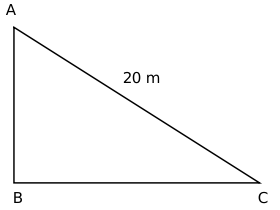BC is ground and it is given that $\angle\ ACB\ =30^{\circ\ }$
Actual length of the tree = AB + AC
$\sin30^{\circ\ }=\frac{AB}{AC}$
AB = 20/2 = 10 cm
Length of the tree = AB + AC = 10 + 20 = 30 cm

Question 6: $ABC$ is a triangle, $\angle B=60^\circ, \angle C=45^\circ$, BC is produced to / extended till D so that $\angle ADB=30^\circ$, then given $\sqrt{3}=\frac{19}{11}$ and $BC \times CD=3^{4}\times 2^{3}\times\frac{11}{19}$, what will be the square of the altitude from A to BC?

a) 144

b) 324

c) 484

d) 1254

Solution: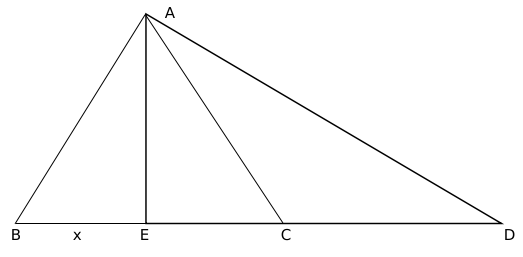AE is altitude from A on BC. $\angle\ AEC\ =\ 90^{\circ\ }$

It is given,

$\angle\ ABC\ =\ 60^{\circ\ },\ \angle\ ACB\ =\ 45^{\circ\ }$

In triangle ABE,

AE = $\sqrt{\ 3}x$

As $\angle\ EAC\ =\angle\ ECA$, EC = $\sqrt{\ 3}x$

In triangle AED, $\angle\ EAD\ =\ 60^{\circ\ }$

Therefore, CD = $3x-\sqrt{\ 3}x$

It is given,

$BC\ \cdot\ CD\ =\ \frac{3^4.2^3.11}{19}$

$x\left(\sqrt{\ 3}+1\right)\sqrt{\ 3}\left(\sqrt{\ 3}-1\right)x\ =\ \frac{3^4.2^3.11}{19}$

$x\ =\ \frac{3^2.2.11}{19}$

AE = 9*2 = 18

$AE^2$ = 324

Question 7: ABCD is a rectangle in the clockwise direction. The coordinates of A are (1,3) and the coordinates of C are (5,1), the coordinates of vertices B and D satisfy the line $y=2x+c$, then what will be the coordinates of the mid-point of BC.

a) $(\frac{5}{2},\frac{7}{2})$

b) $(\frac{9}{2},\frac{5}{2})$

c) $(\frac{9}{5},\frac{7}{2})$

d) $(3,2)$

Solution: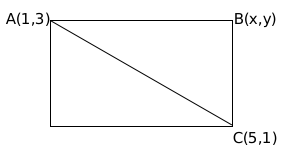Midpoint of AC passes through the line y = 2x + c

Midpoint of AC = (3,2)

2 = 6 + c

c = -4

Line passing through B is y = 2x – 4

(slope of AB)(slope of BC) = -1

$\left(\ \frac{\ y-1}{x-5}\right)\left(\ \frac{\ y-3}{x-1}\right)=-1$

Substituting y = 2x – 4 and solving, we get

B(2,0) or B(4,4)

B cannot lie on X-axis. Therefore, co-ordinates of B = (4,4)

Midpoint of BC = $\left(\frac{9}{2},\frac{5}{2}\right)$

Question 8: In a figure, $\triangle ABC$ is a right angled triangle at $C$, semicircles are drawn on $AC$, $BC$ and $AB$’s diameter. Find the area of the shaded region.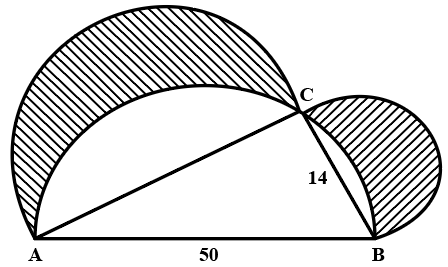Note: Figure not as per scale

a) 336

b) 676

c) 196

d) 556

Solution:

Area of shaded region = area of semicircle on BC + area of semicircle of AC – area of unshaded semicircle regions
Area of unshaded semicircle regions = area of semicircle on AB – area of triangle ABC
Given,
AB = 50, BC = 14
In triangle ABC, $AC^2+BC^2 = AB^2$
Solving we get,
AC = 48
Area of unshaded semicircle regions = $\pi\ \left(25\right)^2\$ – $\frac{1}{2}\times\ 48\times\ 14$ = $625\pi\ -336$
Area of shaded region = $576\pi\ +49\pi\ -625\pi\ +336$ = 336

Question 9: In the figure (not drawn to scale) given below, if $AD=CD=BC$ and angle $BCE = 81^\circ$, how much is the value of angle $DBC$?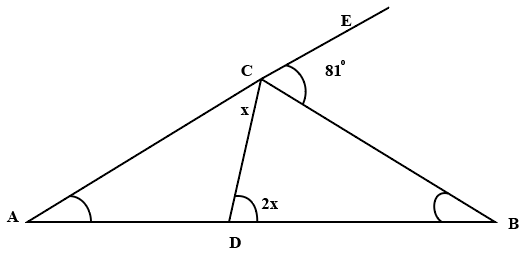a) $27^\circ$

b) $33^\circ$

c) $54^\circ$

d) $66^\circ$

Solution:

Given,
AD = DC, this implies angle ACD = angle DAC = x
DC = BC, this implies angle CDB = angle CBD = 2x
$\angle\ DCB$ = 180 – x – 81 = 99 – x
In triangle BCD, sum of the angles is 180 degrees
99 – x + 2x + 2x = 180
3x = 81
x = 27
$\angle\ DBC$ = 2x = 54 degrees

Question 10: A triangular park named ABC, is required to be protected by green fencing. The length of the side BC is 293. If the length of side AB is a perfect square, the length of the side AC is a power of two (2), and the length of side AC is twice the length of side AB. Determine how much fencing is required to cover the triangular park.

a) 1079

b) 1024

c) 1096

d) 1061

Solution:

ABC is a triangle and BC =  293
Let the length of AB = $x^2$
The length of AC = $2^y$
AC = 2AB
$2^y = 2.x^2$
$2^y-1 = x^2$
$2^y-1$ is a perfect square when y is odd.
Sum of any two sides of a triangle should be larger than the third side. Therefore,
$2^y + x^2$ > 293, 293 + $2^y$ > $x^2$ and 293 + $x^2$ > $2^y$
y > 8
If y = 9, AC = 512, x = 16; satisfies above equations.
If y = 11, AC = 2048, x = 32; doesn’t satisfy above equations.
Therefore, AB = 256 and AC = 512
Fencing required = 256 + 512 + 293 = 1061

Question 11: The angle of elevation of a glider from the deck of ship 25 meters above the sea surface is $30^\circ$ nd the angle of depression of the reflection of glider in the sea is $75^\circ$. Find the approximate height of the glider from the sea surface. $\sqrt{3}=\frac{19}{11}$

a) 46

b) 34

c) 29

d) 52

Solution: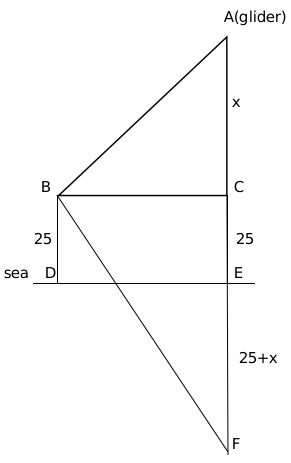It is given, angle ABC = 30 degrees and angle FBC = 75 degrees

$\tan\ 30^{\circ\ }=\frac{x}{BC}$

$BC\ =\ x\sqrt{\ 3}$

$\tan75^{\circ\ }=\ \frac{\ 50+x}{x\sqrt{\ 3}}$

$2+\sqrt{\ 3}\ =\ \frac{\ 50+x}{x\sqrt{\ 3}}$

Solving we get x = 9.1

Height of glinder from sea surface = 25+x $\approx\$ 34

Question 12: Three small identical circles are inscribed inside an equilateral triangle with length $10\sqrt{3}$ cm as shown in the figure. The radius of each small circle is 2 cm A big circle touches these three circles as shown in the figure. Find the ratio of the area of the big circle with that of the area of the small circle. (figure not as per scale)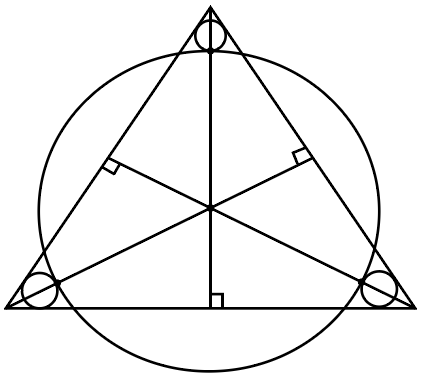a) 4:1

b) 2:1

c) 3:1

d) 5:1

Solution:

Height of triangle = $\left(10\sqrt{3}\right)\times\ \left(\frac{\sqrt{3}}{2}\right)=15$

Distance between the centroid of the triangle and the vertex= $15\times\ \frac{2}{3}=10$

Since in-radius is one-third of the height of the triangle

therefore, length of the line from vertex to the point where small circle and big circle touch each other = $2\times3=6$

Radius of the bigger circle = 10 – 6 = 4cm

Ratio of areas will be square of the ration of radius which is 4:1.

Question 13: There are 12 points in a two-dimensional plane with following coordinates: Points A, B, C, D, E,F, G have coordinates (1, 0), (2, 0), (3, 0), (4, 0), (5, 0), (6, 0) and (7, 0) respectively. Points H, I, J havecoordinates (1, 1), (2, 2) and (3, 3) respectively. Points K, L have coordinates (4, -2) and (5, -3)respectively. The number of circles possible with these points are?

a) 179

b) 158

c) 168

d) 147

Solution:

A, B, C, D, E, F, G all lie of a single line

H, I, J all lie on another single line

K, L lie on another single line

If we are given three points which are not collinear than we can draw a circle from these three points

Question 14: Evaluate $\left[\cos^2 \left(\frac{\pi}{32}\right) + \cos^2 \left(\frac{3 \pi}{32}\right) + \cos^2 \left(\frac{5 \pi}{32}\right) + … + \cos^2 \left(\frac{15 \pi}{32}\right)\right] – \left[\sin^2 \left(\frac{\pi}{16}\right) + \sin^2 \left(\frac{2 \pi}{16}\right) + … + \sin^2 \left(\frac{7 \pi}{16}\right)\right]$

a) 1

b) $\frac{1}{2}$

c) $\frac{1}{3}$

d) 2

Solution:

$\cos\left(A\right)\ =\sin\left(\frac{\pi}{2}-A\right)$

$\sin A=\cos\left(\frac{\pi}{2}-A\right)$

$\cos\left(\frac{\pi}{32}\right)=\sin\left(\frac{\pi}{2}-\frac{\pi}{32}\right)=\sin\left(\frac{15\pi}{32}\right)$

$\sin^2\left(A\right)+\cos^2\left(A\right)=1$

So the series simplifies to (1+1+1+1)-(1+1+1+1/2)

therefore value of series is 1/2

Question 15: If area of the adjacent faces of a cuboid is given as p, q and r respectively and the volume isgiven as ‘V’ then the square of the volume will be

a) $pqr$

b) $\frac{qr}{p^2}$

c) $\frac{(pq)^2}{r^2}$

d) $(pqr)^2$

Solution:

Let the length, breadth and height of the cubhoid be l, b and h respectively.

Therefore of adjacent faces will be lb, bh and hl.

If lb= p, then bh= q and hl=r.

Volume (V) = lbh

$V^2=\left(lbh\right)^2$

or, $V^2=pqr$

Question 16: Two towers 10 meters apart, are 4 m and 6 m high respectively. What will be the height of pointof intersection of lines joining the top of each tower to the bottom of opposite tower?

a) 2.2 meters

b) 1.5 meters

c) 5.5 meters

d) 2.4 meters

Solution: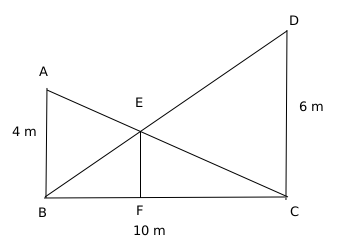Let AB and CD be the towers of length 4m and 6m respectively.

Let the length of BF be x therefore, the length of FC will be 10-x.

Triangle BEF is similar to triangle BDC

So, $\frac{EF}{DC}=\frac{BF}{BC}=\frac{BE}{BD}$

or, $\frac{EF}{6}=\frac{x}{10}=\frac{BE}{BD}$

Therefore, $EF=\frac{3x}{5}$

Similarly, triangle EFC is similar to triangle ABC

So, $\frac{EF}{AB}=\frac{CF}{BC}=\frac{EC}{AC}$

or, $\frac{EF}{4}=\frac{10-x}{10}=\frac{EC}{AC}$

or, $EF=\ \frac{2\left(10-x\right)}{5}$

Therefore, $\frac{2\left(10-x\right)}{5}=\frac{3x}{5}$

or, $x=4$

EF= $\frac{3x}{5}=2.4\ m$

Question 17: For maintaining social distancing due to covid situation, Rohan, Sohan and Rahul are sittingequidistantly, at a distance of 3 meter in a triangular formation. Priya came and sat in between Sohan andRahul such that distance between Priya and Sohan is half the distance between Rahul and Priya. What willbe the distance between Priya and Rohan in meter?

a) $\sqrt{5}$

b) $\sqrt{6}$

c) $\sqrt{7}$

d) $\sqrt{8}$

Solution:

Considering the data given, Rohan, Sohan, and Rahul are sitting in the shape of an equilateral triangle with sides 3m each.

Since Priya’s distance from Sohan is half her distance from Rahul, she is sitting at a distance of 1 m from Sohan.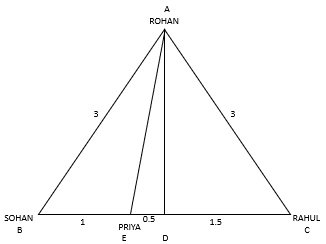Draw a perpendicular AD on BC.

In $\triangle$ ADC, using the Pythagoras theorem,

$AC^2=AD^2+DC^2$

$3^2=AD^2+1.5^2$

$AD=\frac{3\sqrt 3}{2}$

In $\triangle ADE, using the Pythagoras theorem,$AE^2=AD^2+DE^2AE^2=\frac{27}{4}+\frac{1}{4}=7AE=\sqrt 7$Hence, the answer is option C. Question 18: Let ABCD be a parallelogram. The lengths of the side AD and the diogonal AC are 10cm and 20cm, respectively. If the angle$\angle ADC$is equal to$30^{0}$then the area of the parallelogram, in sq.cm is a)$\frac{25(\sqrt{5}+\sqrt{15})}{2}$b)$25(\sqrt{3}+\sqrt{15})$c)$\frac{25(\sqrt{3}+\sqrt{15})}{2}$d)${25(\sqrt{5}+\sqrt{15})}$18) Answer (B) Solution: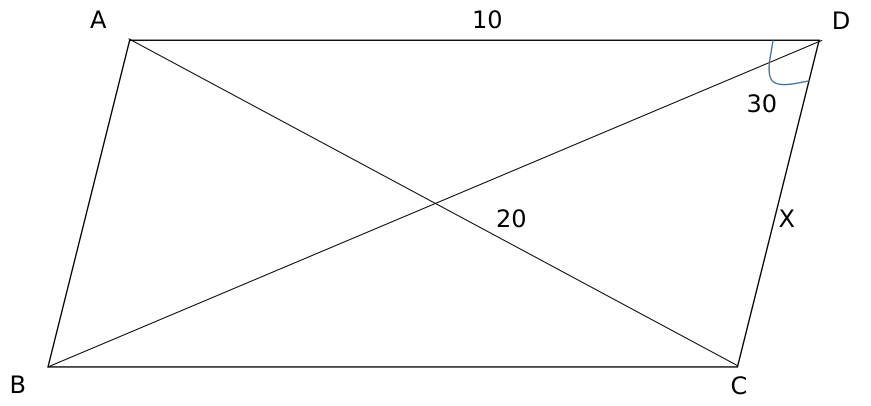Applying cosine rule in triangle ACD,$100+X^2-2\times\ 10\times\ X\cos30=400X^2-10X\sqrt{\ 3}-300=0$Solving, we get X =$\left(\frac{10\sqrt{\ 3}+10\sqrt{\ 15}}{2}\right)$Hence, area = 10Xsin 30 =$\frac{\left(\frac{10\sqrt{\ 3}+10\sqrt{\ 15}}{2}\right)10}{2}$=$25(\sqrt{3}+\sqrt{15})$Question 19: If a triangle ABC,$\angle BCA=50^{0}$. D and E are points on$AB$and$AC$, respectively, such that$AD=DE$. If F is a point on$BC$such that$BD=DF$, then$\angle FDE$, in degrees, is equal to a) 72 b) 80 c) 100 d) 96 19) Answer (B) Solution: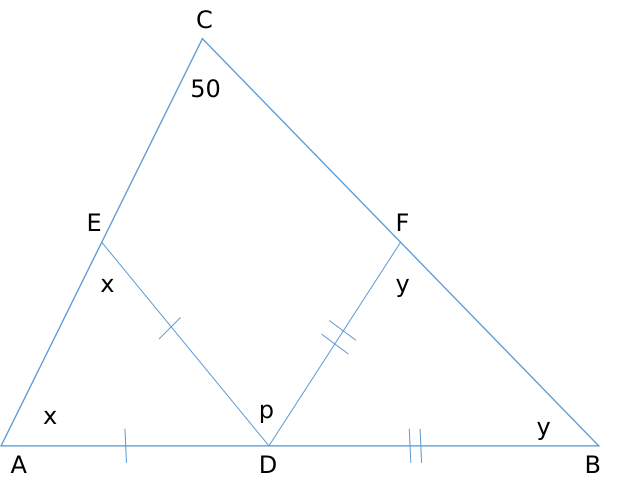We need to find out p. Angle ADE = 180 – 2x Angle BDF = 180 – 2y Now, 180 – 2y + p + 180 – 2x = 180 [Straight line = 180 deg] p = 2x + 2y – 180 Also, x + y + 50 = 180 [Sum of the angles of triangle = 180] x + y = 130 p = 260 – 180 = 80 degrees. Question 20: A park is shaped like a rhombus and has area 96 sq m. If 40 m of fencing is needed to enclose the park, the cost, in INR, of laying electric wires along its two diagonals, at the rate of ₹125 per m, is 20) Answer: 3500 Solution: We can say 40m is the perimeter of the park so side of rhombus = 10 Now$\frac{1}{2}\times\ d_1\times\ d_2\ =\ 96$so we get$\ d_1\times\ d_2\ =\ 192$(1) And as we know diagonals of a rhombus are perpendicular bisectors of each other : so$\ \frac{d_1^2}{4}+\ \frac{d_2^2}{4}=\ 100$so we get$\ d_1^2+\ d_2^2=\ 400$(2) Solving (1) and (2) We get$d_1=12$and$d_2=16\$
Now the cost, in INR, of laying electric wires along its two diagonals, at the rate of ₹125 per m, is= (12+16)(125) =3500